GET THE APP

New PVT Correlations Based on Libyan Crudes for Predicting Fluid Physical Properties | OMICS International
Oil & Gas Research

Like us on:

# New PVT Correlations Based on Libyan Crudes for Predicting Fluid Physical Properties

Khazam M*, Shlak M and Alkhaboli M

Department of Petroleum Engineering, University of Tripoli, Libya

*Corresponding Author:
M Khazam
Department of Petroleum Engineering
University of Tripoli, Tripoli, Libya
Tel.: +218 21-4627901
E-mail: [email protected]

Received Date: November 15, 2016; Accepted Date: November 22, 2016; Published Date: November 28, 2016

Citation: Khazam M, Shlak M, Alkhaboli M (2016) New PVT Correlations Based on Libyan Crudes for Predicting Fluid Physical Properties. Oil Gas Res 2:122. doi: 10.4172/2472-0518.1000122

Copyright: © 2016 Khazam M, et al. This is an open-access article distributed under the terms of the Creative Commons Attribution License, which permits unrestricted use, distribution, and reproduction in any medium, provided the original author and source are credited.

Visit for more related articles at Oil & Gas Research

#### Abstract

PVT properties are very important in reservoir and production engineering analyses such as material balance calculations, well testing, reserve estimation, inflow performance, production operations and design of surface facilities. New empirical PVT correlations have been developed for Libyan crudes with reliable degree of accuracy. These include; bubble point pressure (Pb), oil formation volume factor (Bo), gas solubility (Rs), stock tank oil molecular weight (Mwt), dead oil viscosity (μod), saturated oil viscosity (μob), under-saturated oil viscosity (μo), and oil compressibility (Co). Around 300 PVT samples collected exclusively from Libya, mainly Sirte, Ghadames and Murzuq basins, were used in our study to develop the above PVT correlations and covered wide range of API gravity (26 to 51°API) and reservoir temperature (100 to 313°F) normally found in Libyan reservoirs. Minitab regression tool was extensively used in our study to develop the PVT correlations and to statistically appraise them against the industry published correlations. The new proposed PVT correlations have demonstrated much better performance compared to the industry published correlations when tested for Libyan crudes. Also, Artificial Neural Network (ANN) models have been developed for Libyan PVT properties predictions. The models show acceptable accuracy and generally are more accurate than the empirical correlations.

#### Keywords

PVT properties; Empirical correlations; Artificial neural network

#### Nomenclature

API: Stock-tank oil gravity from flash separation, °API;

Bob: Oil formation volume factor at bubble-point pressure;

Mwt: Molecular weight of stock-tank oil;

Pb: Bubble-point pressure, (Psia);

Ps: Actual separator pressure, (Psia);

Rs: Total producing gas/oil ratio from flash separation, SCF/STB;

T: System temperature, °F;

Ts: Actual separator temperature, °F;

Yg: Gas mole fraction;

μob: Saturated oil Viscosity (cP);

μo: under saturated oil Viscosity (cP);

CO: Isothermal Compressibility Coefficient (psi-1);

ARE: Average relative error (%);

AARE: Absolute average relative error (%);

ANN: Artificial Neural Network;

γo: Specific gravity of stock-tank oil from flash separation, (water=1.0);

γg: Average specific gravity of total surface gases from flash sep. (air=1.0);

γgs: Gas gravity from separator conditions of 100 psig, (air=1.0);

Kuop: Watson paraffin city characterization factor.

#### Data Points and Statistical Indicators

All the data points used in this study are exclusively obtained from Libya, mostly for reservoirs from Sirte, Ghadames, Murzuq and offshore basins. On average basis, around 300 PVT sample data points were initially obtained for each property. The actual number of data points after excluding incompetent measurement and screening for unreasonable values are shown in Table 1 below. This table describes the range and number of experimental data points used in our study. Wider range of fluid properties is used in developing our PVT correlations making them much more representative for the crudes of Libya. In order to evaluate the predictions of the PVT correlations in terms of their accuracy, or their dispersion, or even their representation of the suggested model to the measured data, we have used the following common statistical indicators:

• Average per cent relative error

• Average absolute per cent relative error

• Standard Deviation

• R squared

Property Range (Min-Max) Number of PVT Data Points
Oil FVF (rbbl/stb) (1.034-2.22) 312
Oil Gravity (26-51) -
Temparature (ºF) (100-313) -
Gas Solubility (scf/stb) (48-3583) 285
Gas Gravity (air = 1) (0.6878-1.677) -
Saturated oil viscosity (cP) (0.0908-2.22) 103
Under saturated viscosity (cP) (0.0851-6.056) 300
Oil Compressibility (Psi^-1) (6.11E-06-3.91E-05) 340
Bubble point (Psia) (128-6344) 285

Table 1: Range of Libyan PVT Properties and Number of Samples.

It should be pointed out that no individual statistical indicator should be thought upon as the ultimate gauge that can decide which correlation is better. Choosing the best correlation was assessed with many statistical indicators and was looked upon as an optimization process where each statistical indicator complements the others. Also scatter plots were constructed along with Pearson correlation in order to evaluate the relationships between variables to help in the process of formulations the new correlations. Residual plots, 3D plots and 45 degree plots were also constructed, beside the statistical indicators, in order to evaluate and visualize the suggested models compared to former studies.

#### Oil Formation Volume Factor Correlation

Performing multiple linear regression using Minitab after adjusting the following variables; X1=Rs, X2=Rs/API, X3=Rs/?g, X4=Rs2/API2, and X5=T-Rs. The best regression analysis results were obtained by using the following oil FVF relation: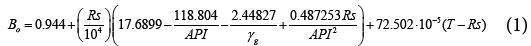Where,

Bo=Oil FVF (rbbl/stb)

γg=Average specific gravity of total surface gases from flash separation (air=1)

Rs=Gas solubility (scf/stb)

T=System temperature, (°F)

API=Stock-tank oil gravity from flash separation (°API)

The first term is a constant that is close to unity followed be a second term that fully depicts the effect of API as well as Gas gravity and the effect of Rs. The third term partially depicts the Rs effect while fully represents the temperature effect. Table 2 below describes the statistical indicators of different industry published Bo correlations with our new formulated Bo correlation for Libyan crudes. As indicated from the this table, the new proposed correlation in this study has relatively better accuracy over the published industry correlations. Taking into account the sensitivity of Bo in reserve estimations and simplicity and accuracy of our correlation, therefore, the new proposed correlation can be adopted to best represent the Libyan crude oils. Figure 1 presents the incremental impact of X variables on the increase in R-squared value. As it is observed from the figure how much did each variable increase the R-squared value of the correlation? The gas solubility (Rs) is the most contributing factor to increase of R-squared value. The new developed correlation yielded a close to accurate prediction of the experimental Bo with R-squared equal to 97.86%, as shown in Figure 2 in the green side. Figure 3 below provides a better understanding of the reliability of the proposed new Bo correlation in compression to the industry published correlations using 45 line graphs. As one can see how the points are within a close fit of the measured Bo for our new correlation and Khazam  correlation which was developed for the same Libyan crude but with less data samples than those used in our new correlation.

Correlations ARE, % AARE, % SD, % R^2, %
Standing (1947) -1.07 2.90 3.99 95.16
Vazquez & Beggs (1980) 2.29 3.65 4.04 93.03
Glaso (1980) 1.68 3.29 3.64 96.77
AL Marhoun (1988) 1.78 3.00 3.32 96.41
Khazam (1995) -0.12 2.26 3.27 97.74
New Correlation -0.10 2.23 3.22 97.86

Table 2: Comparison between the new correlation and industry published correlations.

#### Bubble Point Pressure Correlation

Different approach has been followed in which the number of independent variables were reduced to two,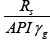and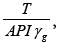making use of curve fitting tool to perform non-linear regression, the following correlation was obtained: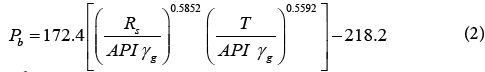Where,

Pb=Bubble point pressure or saturation pressure (psia)

γg=Average specific gravity of total surface gases from flash separation (air=1)

Rs=Gas solubility (scf/stb)

T=System temperature, (°F)

API=Stock-tank oil gravity from flash separation (°API)

Table 3 below describes the statistical indicators of different industry published Pb correlations with our new formulated Pb correlation for Libyan crudes. The newly constructed correlation does not achieve the aimed statistical results. It slightly falls behind the Khazam correlation in terms of (AARE and SD), but it has better R2 prediction over all former published correlations. Khazam  correlation, developed in 1995 for Libyan crudes with less data points used in our study, has the same form of Standing  correlation with different empirical parameters that adjusted to better fit the Libyan crudes data and it has the following form: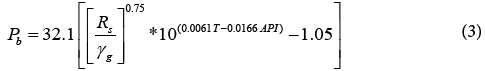Correlation ARE, % AARE, % SD, % R^2, %
Standing (1947) 6.55 14.23 17.27 92.90
Lasater (1958) 8.91 15.41 17.46 92.90
Vazquez & Beggs (1980) -2.86 14.16 17.93 92.90
Glaso (1980) 3.67 18.09 23.17 92.60
AL Marhoun (1988) 11.61 18.17 18.71 88.70
Khazam (1995) 3.16 12.94 16.66 93.70
New correlation -1.36 13.31 21.02 95.90

Table 3: Statistical analyses of Pb correlations.

Figure 4 shows a comparison between the Pb calculated with the new correlation and the measured Pb data. Standard deviation was determined to be about 21%, average relative error equal to -1.36%, and the average absolute relative error determined to be about 13.3%. No indications of abnormal or odd residual values that might be considered as large residuals, where the residual is the difference between the measured value and the predicted value of the Pb. The tested data for our new correlation are all laying within the trend of measured data. Figure 5 shows the cross plot of the new correlation and how the points are within a close fit of the measured Pb for our new correlation. Figure 6 below provides a better understanding of the reliability of the industry Pb published correlations using 45 line graphs.

As one can see that Khazam  correlation is within a close fit of the measured Pb, since was developed for the same Libyan crude data, and is quite comparable with the new proposed form, and both of them have relatively better performance than other published correlations when tested for Libyan crudes.

Gas solubility correlation

The conventional approach was followed, which is to solve the saturation pressure correlation for Rs, which gives the following correlation: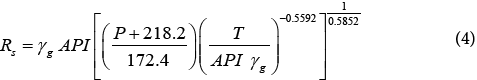Where “P” is defined as system pressure at saturation condition (psia). Table 4 below describes the statistical indicators of different industry published Rs correlations with our new formulated Rs correlation for Libyan crudes. Figure 7 shows the cross plot of the new correlation and how the points are within a close fit of the measured Rs for our new correlation, especially at higher solution GOR values, volatile type of oils. Standard deviation was determined to be about 24%, average relative error equal to -1.45%, and the average absolute relative error determined to be about 16.8%. The tested data for our new correlation are all laying within the trend of measured data.

Correlations ARE, % AARE, % SD, % R^2, %
Standing (1947) -11.86 19.50 23.94 90.90
Lasater (1958) -29.00 32.39 32.41 73.60
Vazquez & Beggs (1980) 17.73 22.78 19.42 76.80
Glaso (1980) -8.42 21.04 27.74 89.80
AL Marhoun (1988) -26.94 34.52 39.65 65.60
Khazam (1995) -7.41 17.91 22.90 88.90
New correlation -1.45 16.84 24.09 91.00

Table 4: Statistical analyses of Rs correlations.

Many models for dead oil viscosity correlation have been tried and tested based on the temperature and API as correlating parameters, but we were unable to reach the aimed statistical results. Howevre, when we have integrated Khazam  molecular weight correlation instead of the API gravity, as correlating parameter, we have achieved better model results. The dead oil viscosity correlation was derived based on 274 data point samples and has the following final form: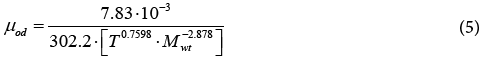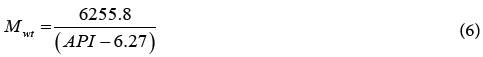where,

Mwt=Calculated stock-tank molecular weight (lb/gmol)

T=System temperature, (°F)

Table 5 below describes the statistical indicators of different industry published μod correlations with our new formulated μod correlation for Libyan crudes. Figure 8 below provides a better understanding of the reliability of the proposed new μod correlation in comparison to the industry published correlations using 45 line graphs. As one can see, it is quite difficult to obtain a close fit to the measured μod data, since one main reason behind this is the challenges and levels of viscosity uncertainty measurements between different industry labs. SD for our new correlation was determined to be about 26%, ARE equals to -4.13%, the AARE determined to be about 21%, and the R2 value is 71%. The tested data for our new correlation are all laying within the trend of measured data and no indications of abnormal or odd values predictions. Labedi  correlation has also reliable predictions since was developed for similar crudes, but our correlation tends to have much better predictions at higher viscosity measured values. Other published industry correlations are obviously deviated from the measured Libyan data with noticeable scattered abnormal trends.

Correlation ARE, % AARE, % SD, % R^2, %
Beal’s (1946) 41.81 42.53 21.79 -12.60
Beggs-Robinson (1975) 13.89 26.08 29.07 38.80
Glaso (1980) 36.77 37.11 16.88 5.40
Labedi (1992) 4.17 22.96 28.34 66.90
Kartoatmodjo-Schmidt (1994) 43.75 43.90 17.17 -13.00
Petrosky-Farshad (1995) 28.91 29.97 18.04 24.30
New correlation -4.13 21.00 25.99 71.10

Table 5: Statistical analyses of µod correlations

#### Saturated Oil Viscosity Correlation

The best model that was found to be more accurate was built on the basis that the saturated viscosity is function of (API, Rs and μod). The final obtained form of saturated viscosity correlation is: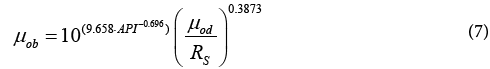where,

μob=Oil viscosity at bubble-point pressure (cP)

Rs=Gas solubility (scf/stb).

API=Oil API gravity (°API)

Table 6 describes the statistical indicators of different industry published μob correlations with our new formulated μob correlation for Libyan crudes. As noticed from Table 6, our new correlation has better accuracy indicators compared to the other industry correlations, with R2 of 85%. Figure 9 shows the comparison between the μob calculated with the new correlation and the measured μob data. The tested data for our new correlation are all lying within the trend of measured data and no indications of abnormal or odd values predictions.

Correlation ARE, % AARE, % SD, % R^2, %
Chew-Connally (1959) -24.82 41.73 56.61 -9.40
Beggs-Robinson (1975) -1.17 31.22 44.23 79.20
Labedi (1992) 10.91 31.00 39.37 67.40
Kartoatmodjo-Schmidt (1994) -26.96 41.78 55.28 55.20
Petrosky-Farshad (1995) -89.00 92.09 125.21 19.70
New correlation -12.31 30.02 45.18 85.30

Table 6: Statistical analyses of µob correlations.

#### Under-saturated Oil Viscosity Correlation

At pressures above the bubble point, the viscosity variation has almost linear relationship with the pressure. Based on several modelling attempts, the final best correlation model we have achieved has the following form: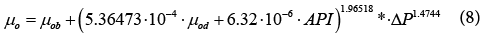where

μob=Viscosity of oil at saturation pressure, (cP)

API=Oil API gravity, (°API)

ΔP=pressure difference above the bubble point pressure, (psi)

It is quite clear that the accuracy predictions of under-saturated oil viscosity is directly linked to the accuracy prediction of saturated oil viscosity. The pressure difference (ΔP) in our correlation is referenced to Pb pressure and in case DP is zero, our correlation will converge to the prediction of μob. This new correlation has achieved higher accuracy performance with R2 of 98.8%, SD of 9.64% and AARE of 5.9%.

#### Isothermal Oil Compressibility Correlation

Isothermal compressibility is used in a wide range of production and reservoir engineering calculations. Some of the reservoir engineering applications for the isothermal compressibility include well testing analysis and material balance calculation for under saturated oil reservoirs. Several correlations [1,3,7,14,15] to calculate oil compressibility have been published and widely used in the oil industry. We have introduced a simple Co correlation with reliable degree of accuracy and it is mainly function of the saturated oil formation volume factor (Bob). The new correlation has the following form: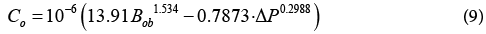where,

Co=Oil compressibility, (psi-1)

Bob=FVF at bubble point pressure, (rbbl/stb)

ΔP=pressure difference above bubble point pressure (psi)

It should be pointed out that Bob which is used in the above equation can be the measured value or the predicted value from the empirical FVF correlations. Figure 10 shows the comparison between the measured and predicted Co with acceptable fit to the measured data. Table 7 below summarises the statistical comparisons with other industry published correlations.

Correlation ARE, % AARE, % SD, % R^2, %
Standing (1947) 14.28 19.15 19.15 52.90
Vazquez-Beggs (1980) 12.48 28.56 34.98 18.60
Labedi (1990) 20.92 21.97 15.66 48.40
Petrosky-Farshad (1995) 18.80 22.38 22.66 53.90
AL Marhoun (2003) 6.90 18.53 21.33 57.90
New correlation -3.49 16.46 19.06 77.60

Table 7: Statistical analysis of oil compressibility correlations.

#### Artificial Neural Network Model

This new computing technique can be defined as massively and highly parallel distributed information processing system that has the ability for recognizing nonlinear relationships within the available data. Neural network resembles the human brain in its function where knowledge is acquired through a learning process; and interneuron connection strengths known as weights are used to store the knowledge The data bank is divided into groups as shown Figure 11, where the learning data are divided into three subsets which are used for training, validation and testing.

#### Development of PVT Neural Network Models

Developed model refers to correlations that predict PVT properties from available field measured variables include oil API gravity, gas gravity, reservoir temperature, viscosity, solution gas oil ratio, bubble point pressure and reservoir pressure, using MATLAB Artificial Intelligent Package (Neural Network Toolbox), a model for each property has been developed to reach a target of best representation of the Libyan crudes. Table 8 and Figure 12 summarize the statistics of ANN’s in comparison with the new developed PVT correlations. As it can be noticed from the below graphs, as well as the statistical indicators, the ANN’s models are slightly better than the new developed empirical PVT correlations. ANN was developed for each correlated PVT property with different numbers of hidden layers. The hidden layers were increased to 3 in the case of oil viscosity and oil compressibility predictions, due to variation in data ranges, which makes the presence of 3 hidden layers better for the accuracy of the models predictions.

PVT Property # of hidden layers # of data used for Training # of data used for verification   R^2, %   SD, %   ARE, %   AARE, %
Bob 2 284 50 98.0 3.12 -0.21 2.22
Pb 2 260 46 97.0 19.90 -2.45 11.57
Rs 2 260 46 92.0 37.40 -0.99 20.23
µod 3 233 41 76.6 25.80 -6.07 19.39
µob 3 104 18 87.0 29.70 -37.18 74.98
µo 1 276 40 99.8 6.78 0.05 4.10
Co 3 321 57 84.0 17.80 -4.40 14.33

Table 8: Number of data used to develop the ANN AND Statistical Results.

#### Conclusions

1. New empirical PVT correlations have been developed for Libyan crudes with reliable degree of accuracy. These include; bubble point pressure (Pb), oil formation volume factor (Bo), gas solubility (Rs), stock tank oil molecular weight (Mwt), dead oil viscosity (μod), saturated oil viscosity (μob), under-saturated oil viscosity (μo), and oil compressibility (Co).

2. Around 300 PVT data point samples from Sirte, Muruzq, Ghadamis, and offshore basins were collected, screened and utilized for our study. These covered wide range of crude oils gravity ranges between (26 to 51°API) and reservoir temperature (100 to 313°F) normally found in Libyan reservoirs.

3. Minitab regression tool was extensively used in our study to develop the PVT correlations and to statistically appraise them against the industry published correlations. The new proposed PVT correlations have demonstrated better performance compared to the industry published correlations tested for Libyan crudes.

4. Artificial Neural Network (ANN) models have been developed for Libyan PVT properties predictions. The models show acceptable accuracy and are marginally accurate than the proposed empirical correlations.

#### Acknowledgment

We highly appreciate and thank the Libyan Petroleum Institute for providing us with the PVT data. Also, we would like to thank the members of Petroleum Engineering Department at the University of Tripoli for their academic support.

#### References

Select your language of interest to view the total content in your interested language

### Article Usage

• Total views: 3472
• [From(publication date):
December-2016 - Dec 08, 2021]
• Breakdown by view type
• HTML page views : 2891Can't read the image? click here to refresh